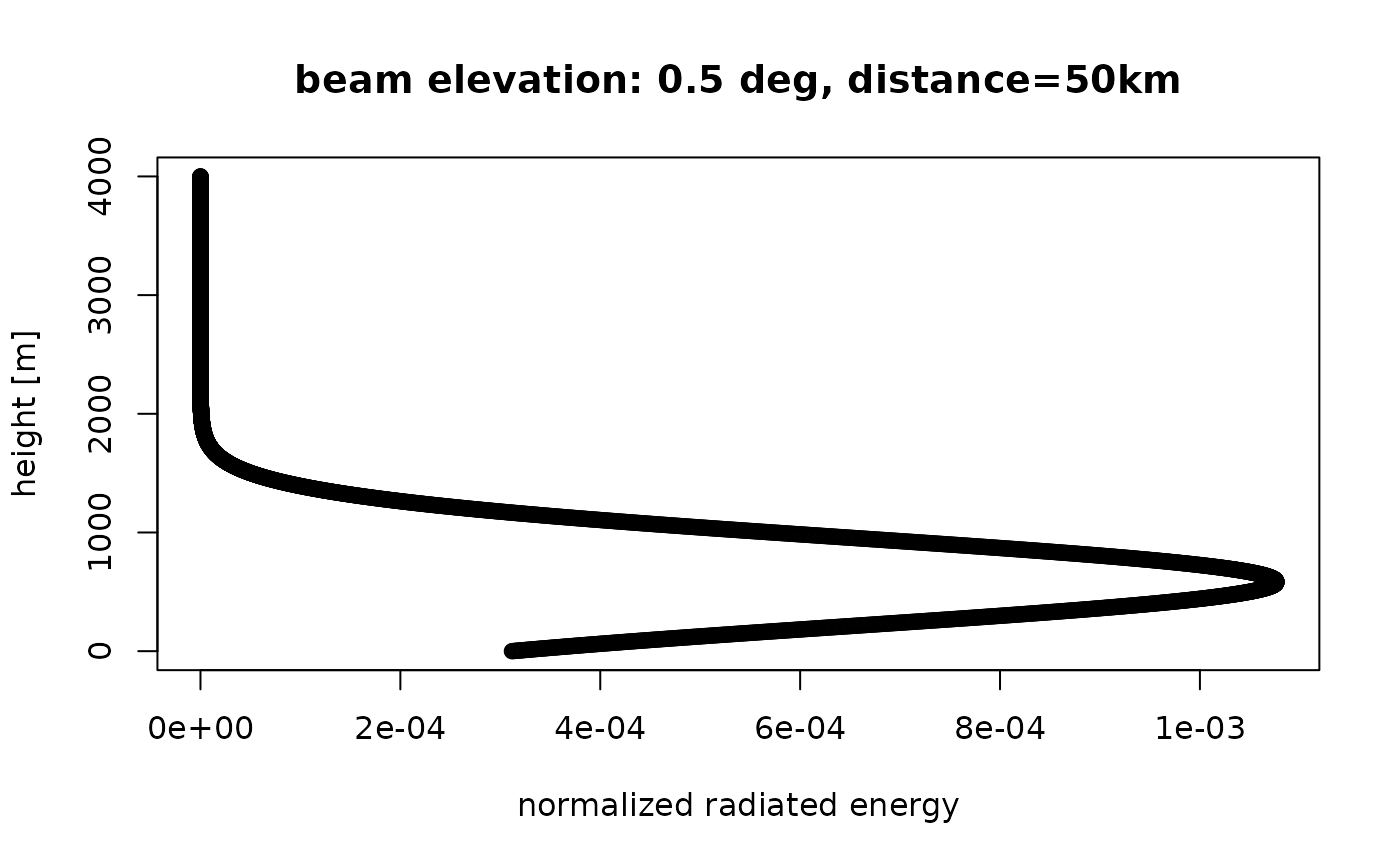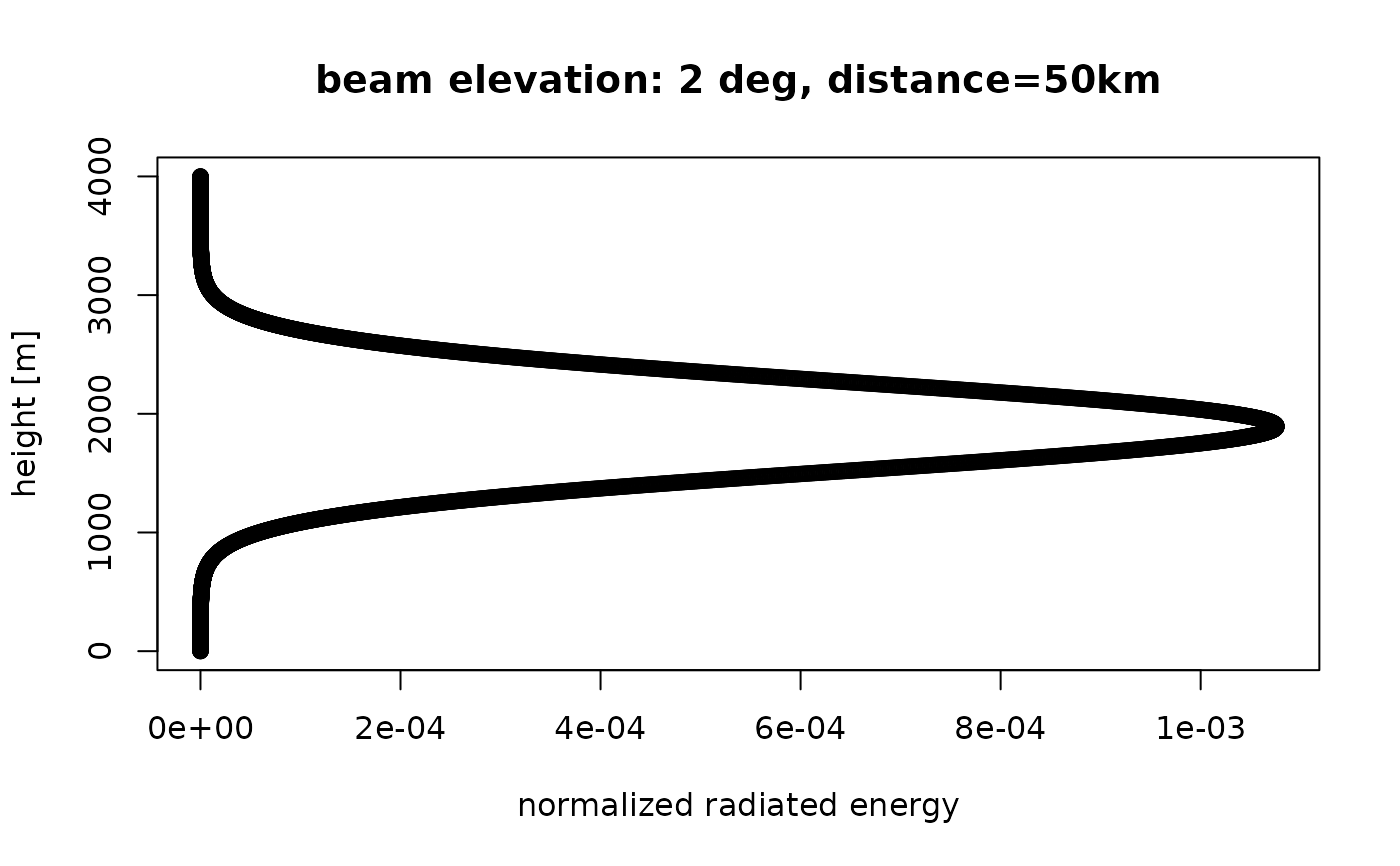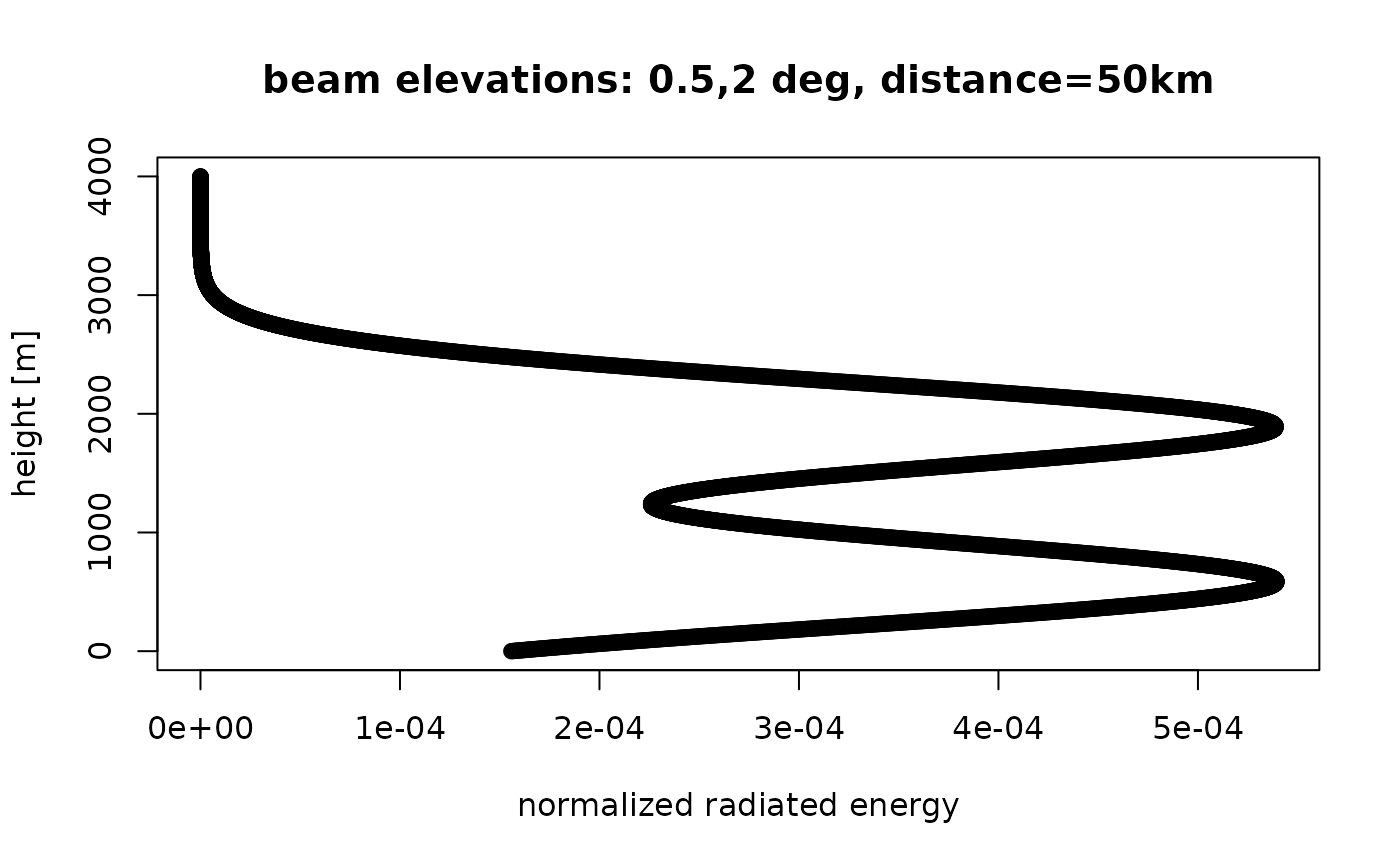Calculate for a set of beam elevations elev the altitudinal normalized distribution of radiated energy by those beams.

beam_profile(
height,
distance,
elev,
antenna = 0,
beam_angle = 1,
k = 4/3,
lat = 35,
re = 6378,
rp = 6357
)

## Arguments

height

numeric. Height in meter.

distance

numeric. Distance from the radar as measured along sea level (down range) in m.

elev

numeric vector. Beam elevation(s) in degrees.

antenna

numeric. Height of the center of the radar antenna in meters

beam_angle

numeric. Beam opening angle in degrees, typically the angle between the half-power (-3 dB) points of the main lobe

k

Standard refraction coefficient.

lat

Geodetic latitude of the radar in degrees.

re

rp

## Value

numeric vector. Normalized radiated energy at each of the specified heights.

## Details

Beam profile is calculated using beam_height and beam_width. Returns a beam profile as a function of height relative to ground level.

Returns the normalized altitudinal pattern of radiated energy as a function of altitude at a given distance from the radar, assuming the beams are emitted at antenna level.

## Examples

# plot the beam profile, for a 0.5 degree elevation beam at 50 km distance from the radar:
plot(beam_profile(height = 0:4000, 50000, 0.5), 0:4000,
ylab = "height [m]", main = "beam elevation: 0.5 deg, distance=50km"
)# plot the beam profile, for a 2 degree elevation beam at 50 km distance from the radar:
plot(beam_profile(height = 0:4000, 50000, 2), 0:4000,